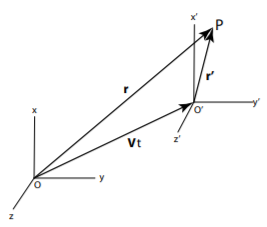$$\require{cancel}$$

# 2.3: Inertial Frames of reference

$$\newcommand{\vecs}{\overset { \rightharpoonup} {\mathbf{#1}} }$$ $$\newcommand{\vecd}{\overset{-\!-\!\rightharpoonup}{\vphantom{a}\smash {#1}}}$$$$\newcommand{\id}{\mathrm{id}}$$ $$\newcommand{\Span}{\mathrm{span}}$$ $$\newcommand{\kernel}{\mathrm{null}\,}$$ $$\newcommand{\range}{\mathrm{range}\,}$$ $$\newcommand{\RealPart}{\mathrm{Re}}$$ $$\newcommand{\ImaginaryPart}{\mathrm{Im}}$$ $$\newcommand{\Argument}{\mathrm{Arg}}$$ $$\newcommand{\norm}{\| #1 \|}$$ $$\newcommand{\inner}{\langle #1, #2 \rangle}$$ $$\newcommand{\Span}{\mathrm{span}}$$ $$\newcommand{\id}{\mathrm{id}}$$ $$\newcommand{\Span}{\mathrm{span}}$$ $$\newcommand{\kernel}{\mathrm{null}\,}$$ $$\newcommand{\range}{\mathrm{range}\,}$$ $$\newcommand{\RealPart}{\mathrm{Re}}$$ $$\newcommand{\ImaginaryPart}{\mathrm{Im}}$$ $$\newcommand{\Argument}{\mathrm{Arg}}$$ $$\newcommand{\norm}{\| #1 \|}$$ $$\newcommand{\inner}{\langle #1, #2 \rangle}$$ $$\newcommand{\Span}{\mathrm{span}}$$

An inertial frame of reference is one in which Newton’s Laws of motion are valid. It is a non-accelerated frame of reference. An inertial frame must be homogeneous and isotropic. Physical experiments can be carried out in different inertial reference frames. The Galilean transformation provides a means of converting between two inertial frames of reference moving at a constant relative velocity. Consider two reference frames $$O$$ and $$O'$$ with $$O'$$ moving with constant relative velocity $${\bf V}$$ at time $$t$$. Figure $$\PageIndex{1}$$ shows a Galilean transformation which can be expressed in vector form.

\begin{equation} \begin{split} \mathbf{r'} & =\mathbf{r}-\mathbf{V}t \\ t' & = t \end{split}\label{eq:2.7}\end{equation}

Equation \ref{eq:2.7} gives the boost, assuming Newton’s hypothesis that the time is invariant to change of inertial frames of reference. Differentiation of this transformation gives

\begin{equation}
\begin{split}
\mathbf{\dot{r}'} & =\mathbf{\dot{r}}-\mathbf{V}\\
\mathbf{\ddot{r}'} & = \mathbf{\ddot{r}}
\end{split}\label{eq:2.8}
\end{equation}

Note that the forces in the primed and unprimed inertial frames are related by \begin{equation}\label{eq:2.9}\mathbf{F}=\frac{d\mathbf{p}}{dt}=m\mathbf{\ddot{r}}=m\mathbf{\ddot{r}'}=\mathbf{F}'
\end{equation}Figure $$\PageIndex{1}$$: Frame $$O'$$ moving with a constant velocity $$V$$ with respect to frame $$O$$ at the time $$t$$.

Thus Newton’s Laws of motion are invariant under a Galilean transformation, that is, the inertial mass is unchanged under Galilean transformations. If Newton’s laws are valid in one inertial frame of reference, then they are valid in any frame of reference in uniform motion with respect to the first frame of reference. This invariance is called Galilean invariance. There are an infinite number of possible inertial frames all connected by Galilean transformations.

Galilean invariance violates Einstein’s Theory of Relativity. In order to satisfy Einstein’s postulate that the laws of physics are the same in all inertial frames, as well as satisfy Maxwell’s equations for electromagnetism, it is necessary to replace the Galilean transformation by the Lorentz transformation. As will be discussed in chapter $$17$$, the Lorentz transformation leads to Lorentz contraction and time dilation both of which are related to the parameter $$\gamma \equiv \frac{1}{\sqrt{1 - (\frac{v}{c})^2}}$$ where c is the velocity of light in vacuum. Fortunately, most situations in life involve velocities where $$v < < c$$; for example, for a body moving at 25 000 mph (11 111 m/s) which is the escape velocity for a body at the surface of the earth, the $$\gamma$$ factor differs from unity by about $$6.8 \times 10^{-10}$$ which is negligible. Relativistic effects are significant only in nuclear and particle physics and some exotic conditions in astrophysics. Thus, for the purpose of classical mechanics usually it is reasonable to assume that the Galilean transformation is valid and is well obeyed under most practical conditions.

This page titled 2.3: Inertial Frames of reference is shared under a CC BY-NC-SA 4.0 license and was authored, remixed, and/or curated by Douglas Cline via source content that was edited to the style and standards of the LibreTexts platform; a detailed edit history is available upon request.